An adiabatic process is one in which no heat is gained or lost by the system. The first law of thermodynamics with Q=0 shows that all the change in internal energy is in the form of work done. This puts a constraint on the heat engine process leading to the adiabatic condition shown below. This condition can be used to derive the expression for the work done during an adiabatic process.The ratio of the specific heats γ = CP/CV is a factor in determining the speed of sound in a gas and other adiabatic processes as well as this application to heat engines. This ratio γ = 1.66 for an ideal monoatomic gas and γ = 1.4 for air, which is predominantly a diatomic gas.

Index

Heat engine concepts

 HyperPhysics***** Thermodynamics R Nave
Go BackIndex

Heat engine concepts

 HyperPhysics***** Thermodynamics R Nave
Go Back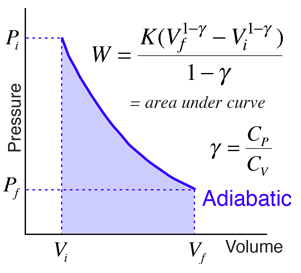For an ideal gas consisting of n = moles of gas, consider an adiabatic process which involves expansion from

 Vi = m3
 to Vf = m3
 at initial temperature Ti = K
Note: If initial volume or temperature are changed, a new value of pressure will be calculated to correspond to the number of moles of gas above.

With the initial volume and temperature specified, the initial pressure is determined by the ideal gas law:

 Using,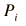= kPa = x10^ Pa
(Note: If the initial pressure value is changed, then a new value of moles will be calculated to be consistent with the initial volume and temperature specified above. If you wish to restrict the number of moles to your supplied value, then enter that value plus values for initial volume and temperature - the required pressure will be calculated. )

The detailed behavior of the pressure and volume depends upon the specific heats of the gas:

 Constant pressure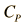= J/mol K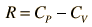Constant volume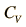= J/mol K
 The specific heats determine the ratio,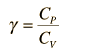=

Note that the value of γ is determined by the gases, the most common values being γair = 1.4 and γmonoatomic gas = 1.66 .

The adiabatic condition can be applied to determine the constant K .=

The work done by the gas can then be determined. If the final volume is less than the initial, then work is done on the gas and the work will be negative.= J = x10^ J

The final pressure for the process can be determined from the adiabatic condition:= kPa = x10^ Pa

and the final temperature can be obtained from the ideal gas law.= K
The specified initial temperature for the work calculation must be in Kelvins, so it must be converted from the other temperature scales.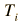= K = °C = °F